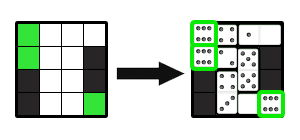시간 제한 메모리 제한 제출 정답 맞은 사람 정답 비율
2 초 256 MB 5 2 2 40.000%

## 문제

Pasha has a board of size $n \times m$. Some cells are colored black, some --- colored green, all remaining cells are colored white. Also, Pasha has a typical domino set consisting of 28 dominoes. He wants to place some of this dominoes on the board in the following way:

• Each domino occupies exactly two neighboring cells;
• Each non-black cell is occupied with exactly one domino;
• There is no black cell occupied with domino;
• The total number of dots on the green cells $g$ is maximized.

You are to write a program to find the maximum possible value of $g$ for the given board.

## 입력

There will be multiple test cases in the input. Each test case starts with two positive integers $n$ and $m$ ($1 \leq n \cdot m \leq 56$). The following $n$ lines contain $m$ characters each. The $i$-th line describes the $i$-th row of the board: 'W' denotes the white cell, 'B' --- the black cell and 'G' --- the green cell. Each board contains at least one green cell. The last test case is followed by a line that contains two zeroes. It must not be processed. There will be no more than $500$ test cases in the input.

## 출력

For each test case output its number and then the maximum value of $g$. If it is impossible to place dominoes in the described way --- output "No solution" instead. Follow the format of the sample output.

## 예제 입력 1

4 4
GWWW
GWWB
BWWB
BWWG
1 3
WGW
0 0


## 예제 출력 1

Case 1: 18
Case 2: No solution


## 힌트

The following image shows how to place dominoes in the first sample: